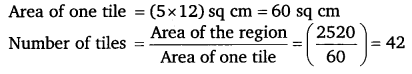# Class 6 Maths NCERT Solutions for Chapter 10 Mensuration Ex – 10.3

## Mensuration

Question 1.
Find the areas of the rectangles whose sides are:

(a) 3 cm and 4 cm
(b) 12 m and 21 m
(c) 2 km and 3 km
(d) 2 m and 70 cm

Solution:
(a) Length of the rectangle = 4 cm
Breadth of the rectangle = 3 cm
= 4 cm x 3 cm
= 12 sq. cm

(b) Length of the rectangle = 21 m
Breadth of the rectangle = 12 m
= 21 m xl2 m
= 252 sq. m.

(c) Length of the rectangle = 3 km
Breadth of the rectangle =2 km
= 3 km x 2km
= 6 sq. km

(d) Length of the rectangle =2m = 2 x l00= 200 cm
Breadth of the rectangle = 70 cm
= 200 cm x 70 cm
= 14000 sq. cm

Question 2.
Find the areas of the squares whose sides are:

(a) 10 cm
(b) 14 cm
(c) 5 m

Solution:
(a) Side of the square = 10 cm
Area = (side)2
= (10)2 sq. cm
= 100 sq. cm

(b) Side of the square = 14 cm
Area = (side)2
= (14)2 sq. cm
= 196 sq. cm

(c) Side of the square = 5 m
Area = (side)2
= (5)2 sq. m = 25sq. m

Question 3.
The length and breadth of three rectangles are as given below:

(a) 9 m and 6 m
(b) 17 m and 3 m
(c) 4 m and 14 m
Which one has the largest area and which one has the smallest?

Solution:
In rectangle (a), Area = (9 x 6) sq. m
= 54 sq. m
In rectangle (b), Area =(3 x 17) sq. m = 51 sq. m
In rectangle (c), Area = (4 x 14) sq. m = 56 sq. m
Clearly, 56 > 54 > 51 i.e., 56 is the largest number and 51 is the smallest number.
∴ The rectangle (c) has the largest area and the rectangle (a) has the smallest area.

Question 4.
The area of a rectangular garden 50 m long is 300 sq m. Find the width of the garden.

Solution:
We use the formula A = l x b, where l is the length and b is the breadth of the rectangle in metre and its area in m2.
Here, A = 300 m2, and l = 50 m
Therefore, 300 =50 x b [ ∵ A = l x b]
or$b=\frac { 300 }{ 50 } =6$
Hence the breadth (width) of the rectangle is 6 m.

Question 5.
What is the cost of tiling a rectangular plot of land 500 m long and 200 m wide at the rate of ₹ 8 per hundred sq. m?

Solution:
Area of rectangular piece of land = 500 x 200 sq. m
= 100000 sq. m
Cost of tiling the land at the rate of ₹ 8 per hundred sq. m
= ₹$\left( 100000\times \frac { 1 }{ 100 } \right)$ = ₹ 8000

Question 6.
A table-top measures 2 m by 1 m 50 cm. What is its area in square metres?

Solution:
Length of table’s top = 2 m
Breadth of table’s top = 1 m 50 cm = 1.50 m
∴ Area = Length x Breadth
= (2 x 1.50) sq m = 3 sq m

Question 7.
A room is 4 m long and 3 m 50 cm wide. How many square metres of carpet is needed to cover the floor of the room?

Solution:
Length of the room = 4 m
Breadth of the room = 3 m 50 cm = 3.50 m
Carpet needed to cover the floor of the room
= Area of the floor of the room
= (4 x 3.50) sq. m = 14 sq. m

Question 8.
A floor is 5 m long and 4 m wide. A square carpet of sides 3 m is laid on the floor. Find the area of the floor that is not carpeted.

Solution: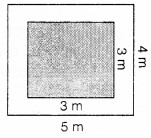Area of the floor of the room = (5 x 4) sq. m
= 20 sq. m
Area of the carpet = (3 x 3) sq. m = 9 sq. m
Area of the floor not carpeted = (20 – 9) sq. m
= 11 sq. m

Question 9.
Five square flower beds each of sides 1 m are dug on a piece of land 5 m long and 4 m wide. What is the area of the remaining part of the land?

Solution:
Area of the piece of land =(5 x 4) sq. m
= 20 sq. m
Area of square flower bed =(1 x 1) sq. m
= 1 sq. m
Area of 5 such flower beds =(5 x 1) sq. m
= 5 sq. m
∴ Area of the remaining part of land
= (20 – 5) sq. m = 15 sq. m

Question 10.
By splitting the following figures into rectangles, find their areas (The measures are given in centimetres).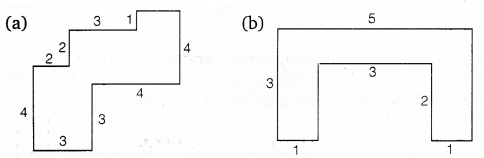Solution:
(a) Let the figure may be divided into rectangles marked as A, B, C etc. Area of rectangle (A) = (3 x 3) sq. cm = 9 sq. cmArea of rectangle (B) = (1 x 2) sq. cm = 2 sq. cm
Area of rectangle (C) = (3 x 3) sq. cm = 9 sq. cm
Area of rectangle (D) = (4 x 2) sq. cm = 8 sq. cm
∴ The total area of the figure = (9 + 2 + 9 + 8) sq. cm = 28 sq. cm
(b) Let the figure may be divided into rectangle marked as shown.Area of rectangle (A) = (2 x 1) sq cm = 2 sq cm
Area of rectangle (B) = (5 x 1) sq cm = 5 sq cm
Area of rectangle (C) = (2 x 1) sq cm = 2 sq cm
∴ Total area of the figure = (2 + 5 + 2) sq cm = 9 sq cm

Question 11.
Split the following shapes into rectangles and find their areas. (The measures are given in centimetres)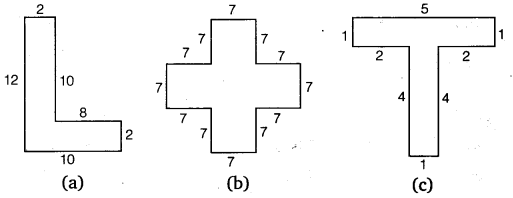Solution:
(a) Splitting the given shape into rectangles as marked A and B.Area of the rectangle (A) = (10 x 2) sq cm
= 20 sq cm
Area of the rectangle (B) = (10 x 2) sq cm
= 20 sq cm
∴ Total area of given shape = (20 +20) sq cm
= 40 sq cm
(b) Splitting the given shape into five squares each of side 7 cm.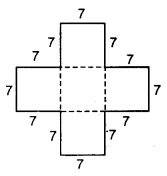Area of the given shape
= 5 x Area of one square of side 7 cm = 5 x (7 x 7) sq. cm
= (5 x 49)sq cm 7
= 245 sq cm
(c) Splitting the given shape into two rectangles named A and B. Area of rectangle (A) = (5 x 1) sq cm = 5 sq cm
Area of rectangle (B) = (4 x 1) sq cm = 4 sq cm
∴ Total area of given shape = (5 + 4) sq cm = 9 sq cm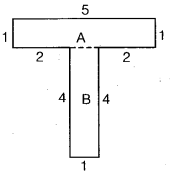Question 12.
How many tiles whose length and breadth are 12 cm and 5 cm, respectively will be needed to fit in a rectangular region whose length and breadth are respectively:

(a) 100 cm and 144 cm
(b) 70 cm and 36 cm

Solution:
(a) Area of the rectangular region
= (100 x 144) sq cm
= 14400 sq cm
Area of one tile = (5 x 12) sq cm
= 60 sq cm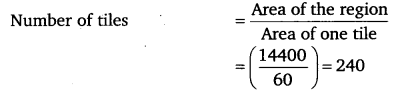(b) Area of the rectangular region
= (70 x 36) sq cm
= 2520 sq cm